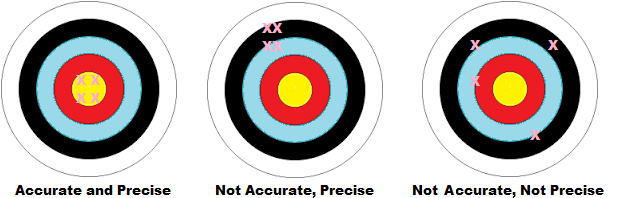# Accuracy and Precision: Definition, Examples

Share on

Design of Experiments > Accuracy and Precision

You can never make exact measurements in an experiment (even the atomic clock isn’t exact: it loses a second every 15 billion years). How far away from the “mark” you are is described by accuracy and how well you measure is described by precision.

## Accuracy

Accuracy is how close you are to the true value. For example, let’s say you know your true height is exactly 5’9″.

• You measure yourself with a yardstick and get 5’0″. Your measurement is not accurate.
• You measure yourself again with a laser yardstick and get 5’9″. Your measurement is accurate.

Note: The true value is sometimes called the theoretical value.

## Precision

Precision is how close two or more measurements are to each other. If you consistently measure your height as 5’0″ with a yardstick, your measurements are precise.

## Accuracy of Analysis and Precision Together

If you are precise, that doesn’t necessarily mean you are accurate. However, if you are consistently accurate, you are also precise.### “More” Precise

If you want to tell which set of data is more precise, find the range (the difference between the highest and lowest scores). For example, let’s say you had the following two sets of data:

• Sample A: 32.56, 32.55, 32.48, 32.49, 32.48.
• Sample B: 15.38, 15.37, 15.36, 15.33, 15.32.

Subtract the lowest data point from the highest:

• Sample A: 32.56 – 32.48 = .08.
• Sample B: 15.38 – 15.32 = .06.

Sample B has the lowest range (.06) and so is the more precise.

## More Examples

• Accurate and precise: If a weather thermometer reads 75oF outside and it really is 75oF, the thermometer is accurate. If the thermometer consistently registers the exact temperature for several days in a row, the thermometer is also precise.
• Precise, but not accurate: A refrigerator thermometer is read ten times and registers degrees Celsius as: 39.1, 39.4, 39.1, 39.2, 39.1, 39.2, 39.1, 39.1, 39.4, and 39.1. However, the real temperature inside the refrigerator is 37 degrees C. The thermometer isn’t accurate (it’s almost two degrees off the true value), but as the numbers are all close to 39.2, it is precise.

## References

Dodge, Y. (2008). The Concise Encyclopedia of Statistics. Springer.
Gonick, L. (1993). The Cartoon Guide to Statistics. HarperPerennial.

CITE THIS AS:
Stephanie Glen. "Accuracy and Precision: Definition, Examples" From StatisticsHowTo.com: Elementary Statistics for the rest of us! https://www.statisticshowto.com/accuracy-and-precision/
---------------------------------------------------------------------------Need help with a homework or test question? With Chegg Study, you can get step-by-step solutions to your questions from an expert in the field. Your first 30 minutes with a Chegg tutor is free!